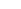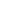# Constructing an Equivalent Regular Grammar from a Regular Expression

## Algorithm

This algorithm is not very straightforward, and may take a while to understand. We will show how to construct a regular grammar from a regular expression, and it is suggested that you try a few simple exercises using RELIC to confirm your results. The method shown here is simply an example on how you can convert a simple regular expression to a regular grammar.

First of all you must remember the definition of a regular expression and the theorem: for any regular expression e, there exists a regular grammar G recognising exactly the language described by e. Refer to the relevant section in the course notes for the proof upon which the algorithm is based.

The basic idea is the following:

• if the regular expression is simply 0, we can show that G, with no production rules, is an equivalent regular grammar.
• if the regular expression is simply 1, we can show that G, with one production rule S(where S is the start symbol), is an equivalent regular grammar.
• if the regular expression is simply a (a being any terminal), we can show that G, with one production rule Sa (where S is the start symbol), is an equivalent regular grammar.
• if the regular expression is of the form e+f, where both e and f are regular expressions, use this algorithm to contruct two regular grammars G1 and G2 equivalent to e and f respectively. Now use regular grammar union on these two grammars to construct a regular grammar G which is equivalent to e+f.
• if the regular expression is of the form ef, where both e and f are regular expressions, use this algorithm to contruct two regular grammars G1 and G2 equivalent to e and f respectively. Now use regular grammar catenation on these two grammars to construct a regular grammar G which is equivalent to ef.
• if the regular expression is of the form e*, where e is a regular expression, use this algorithm to contruct a regular grammar G equivalent to e. Now use regular grammar Kleene star closure to construct a regular grammar G' which is equivalent to e*.
• if the regular expression is of the form e+, where e is a regular expression, use this algorithm to contruct a regular grammar G equivalent to e. Now use regular grammar Kleene plus closure to construct a regular grammar G' which is equivalent to e+.

## Examples

Note that to simplify the presentation, we will describe regular grammars by just stating their production rules. The non-terminal appearing on the left hand side of the first production is assumed to be the start symbol.

### Example 1

Consider the regular expression (a + b)*a. We will now construct a regular grammar for this regular expression. For every terminal symbol a, we create a regular grammar with the rule S \arrow a, start symbol S. We then apply the transformations to these regular grammars, progressively constructing the regular grammar.

First consider the expression a + b. We create two regular grammars:

 S1a and S2b

where S1 and S2 are the start symbols. Clearly, these grammars recognise the regular expressions a and b respectively.

Now, we apply the union transformation for regular grammars to get:

 S3a | b S1a S2b

where S3 is the start symbol. This grammar obviously recognises a + b.

Next, we consider the expression (a + b)*. We already have a regular grammar for (a + b), so now we apply the Kleene star transformation on the regular grammar:

 S4a | b |S3a | b S1aS3 S2bS3

where S4 is the start symbol.

Recall that we need a regular grammar that recognises (a + b)*a. We thus consider again the regular expression a. Again, we create a regular grammar that describes the language:

 S5a

where S5 is the start symbol.

We now construct the catenation of the regular grammar describing (a + b)* together with this one. We simply apply the transformation that catenates two regular grammars, to get:

 S4a | b |S3aS5 | bS5 S1aS3 S2bS3 S5a

where S4 is the start symbol.

This regular grammar is equivalent to the regular expression (a + b)*a.

### Example 2

Consider the regular expression (a + 1)*. We will now construct a regular grammar for this regular expression. This looks almost identical to the first part of the previous example. However we have 1 in the expression, for which we need an equivalent regular grammar. An obvious solution is S.

Now consider the expression a + 1. We create the two regular grammars:

 S1a and S2where S1 and S2 are the start symbols.

Now, we apply the union transformation for regular grammars to get:

 S3a |S1a S2where S3 is the start symbol.

Next, we consider the expression (a + b)*. We already have a regular grammar for (a + 1), so now we apply the Kleene star transformation on the regular grammar. Since the grammar is not-free, we first have to make the grammar-free:

 S4a |S3a S1a

where S4 is the start symbol. Note that we eliminated all production rules originating from S2.

We apply the kleene star transformation to this regular grammar:

 S4a |S3aS4 S1aS4

where S4 is the start symbol.

Notice that this grammar can easily be simplified, but for the sake of the algorithm, we do not simplify it as yet. Note that the regular expression 0 is the empty language.

## Exercises

Construct regular grammars for following regular expressions:

1. a*
2. a + 0
3. (ab)*
4. a* + 1 + b+